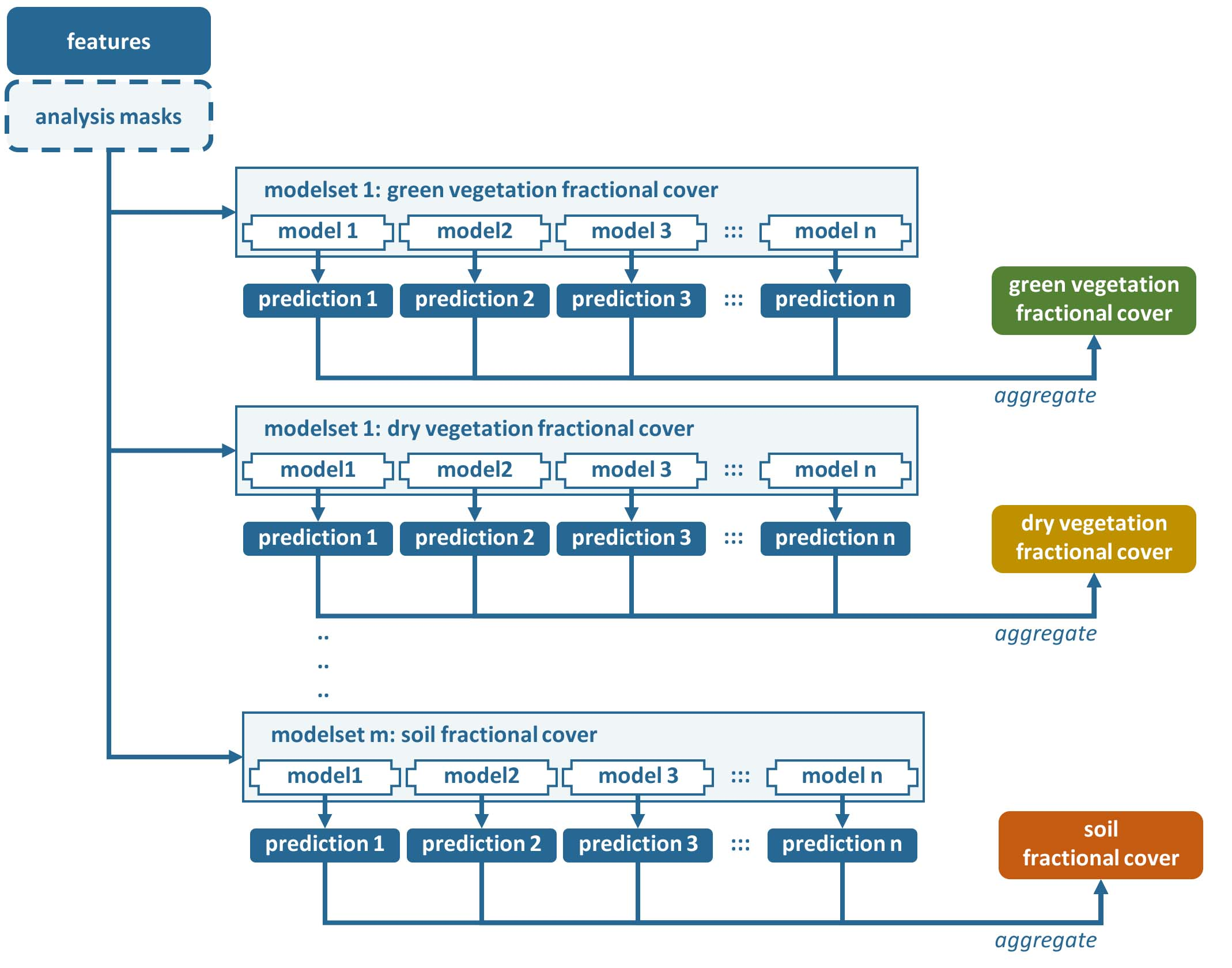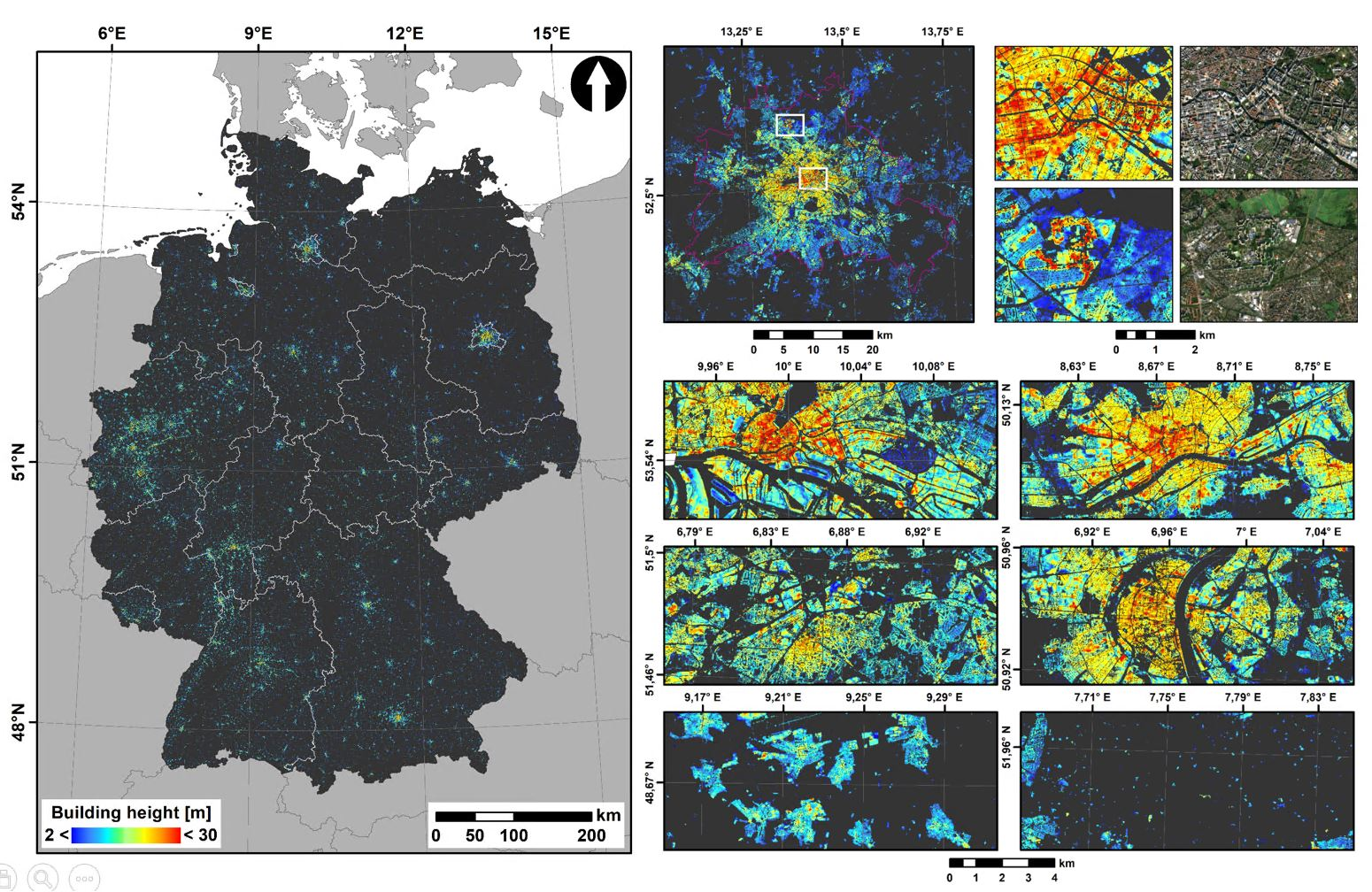# Machine Learning

The Machine Learning submodule generates maps from machine learning predictions. The response variable can either be quantitative or qualitative, i.e. regression or classification. The resulting maps are grouped as highly Analysis Ready Data plus (hARD+), which means they can be directly used to fuel your research questions without any further processing. Typically, this submodule is fed with hARD products, i.e. seamless and gap free aggregate products. hARD products can e.g. be generated by the Time Series Analysis, Level 3 Compositing, Texture Metrics, and Landscape Metrics submodules - or external hARD can be ingested using force-cube. Machine learning models are trained using force-train.Figure Processing workflow of the Machine Learning submodule. In this case, different fractional cover types are the response variables.

The same set of features need to be input, which was used to train the model (force-train). The submodule permits to predict multiple response variables at once, i.e. with the same run. The only constraint is, that each prediction was trained with the same input features, and that the same Machine Learner is used for each model. Available learners are Support Vector Regression, Support Vector Classification, Random Forest Regression, and Random Forest Classification.

A modelset needs to be given for each response variable. Within each modelset, one or multiple models may be input. The number of models may differ from modelset to modelset. A model is a *.xml file generated with force-train.

Within each modelset, the final prediction is generated by aggregating the results of each prediction. For regression, this is the average of the individual model’s predictions. For classification, this is the mode of the individual model’s predictions.

A glimpse of what you get:Figure Building Height prediction for Germany using Support Vector Regression. The model was trained with 3D building models and multi-temporal Sentinel-1+2 A/B time series.